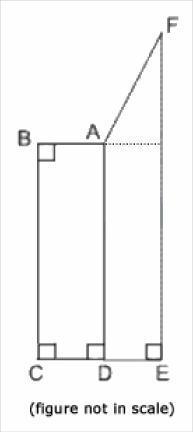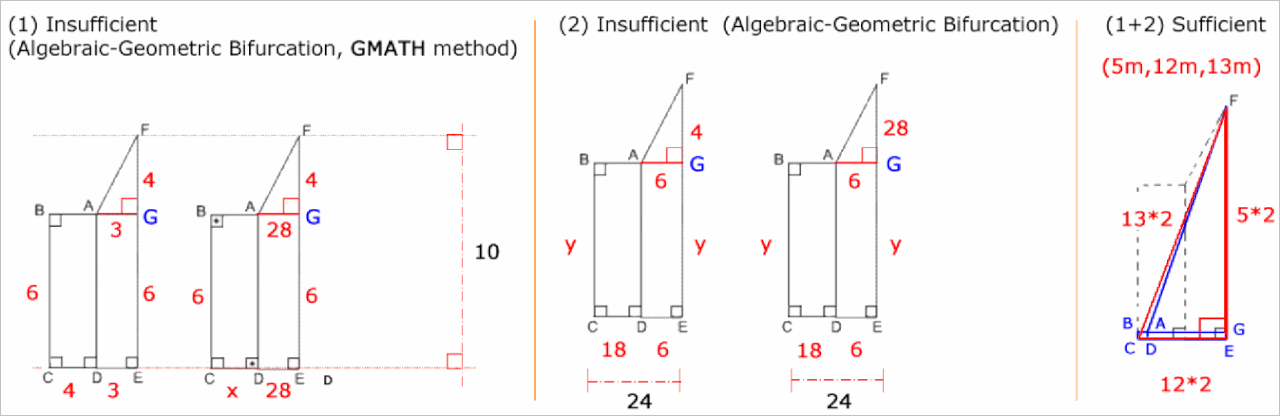• NEW! FREE Beat The GMAT QuizzesHundreds of Questions Highly Detailed Reporting Expert Explanations
• 7 CATs FREE!
If you earn 100 Forum Points

Engage in the Beat The GMAT forums to earn
100 points for $49 worth of Veritas practice GMATs FREEVERITAS PRACTICE GMAT EXAMS Earn 10 Points Per Post Earn 10 Points Per Thanks Earn 10 Points Per Upvote ## If quadrilaterals ABCD and ADEF have the same areas, is AF&a tagged by: fskilnik@GMATH ##### This topic has 1 expert reply and 0 member replies ### GMAT/MBA Expert ## If quadrilaterals ABCD and ADEF have the same areas, is AF&a## Timer 00:00 ## Your Answer A B C D E ## Global Stats Difficult GMATH practice exercise (Quant Class 15)If quadrilaterals ABCD and ADEF have the same areas, is AF > 28 ? (1) EF = 10 (2) CE = 24 Answer: ______(C)__ _________________ Fabio Skilnik :: GMATH method creator ( Math for the GMAT) English-speakers :: https://www.gmath.net Portuguese-speakers :: https://www.gmath.com.br### GMAT/MBA Expert GMAT InstructorJoined 09 Oct 2010 Posted: 1434 messages Followed by: 32 members Upvotes: 59 fskilnik@GMATH wrote: GMATH practice exercise (Quant Class 15)If quadrilaterals ABCD and ADEF have the same areas, is AF > 28 ? (1) EF = 10 (2) CE = 24$${S_{{\mathop{\rm ABCD}\nolimits} }} = {S_{{\rm{ADEF}}}} = {S_{{\rm{ADEG}}}} + {S_{{\rm{AGF}}}}\,\,\,\,\,\left( * \right)$$ $$AF\,\,\mathop > \limits^? \,\,28$$ $$\left( 1 \right)\,\,\left\{ \matrix{ \,{\rm{image}}\,\,{\rm{left}}:\,\,\left( * \right)\,\,4 \cdot 6 = 3 \cdot 6 + {{3 \cdot 4} \over 2}\,\,\,\,\, \Rightarrow \,\,\,\,{\rm{viable}}\,\,\,\, \Rightarrow \,\,\,\,\left\langle {{\rm{NO}}} \right\rangle \hfill \cr \,{\rm{image}}\,\,{\rm{right}}:\,\,\left( * \right)\,\,x \cdot 6 = 28 \cdot 6 + {{28 \cdot 4} \over 2}\,\,\,\,\, \Rightarrow \,\,\,\,{\rm{viable}}\,\,\,\left( {x = {{28 \cdot 8} \over 6} > 0} \right)\,\,\,\, \Rightarrow \,\,\,\,\left\langle {{\rm{YES}}} \right\rangle \hfill \cr} \right.$$ $$\left( 2 \right)\,\,\left\{ \matrix{ \,{\rm{image}}\,\,{\rm{left}}:\,\,\left( * \right)\,\,18 \cdot y = 6 \cdot y + {{6 \cdot 4} \over 2}\,\,\,\,\, \Rightarrow \,\,\,\,{\rm{viable}}\,\,\,\,\left( {y = 1 > 0} \right) \Rightarrow \,\,\,\,\left\langle {{\rm{NO}}} \right\rangle \hfill \cr \,{\rm{image}}\,\,{\rm{right}}:\,\,\left( * \right)\,\,18 \cdot y = 6 \cdot y + {{6 \cdot 28} \over 2}\,\,\,\,\, \Rightarrow \,\,\,\,{\rm{viable}}\,\,\,\left( {y = 7 > 0} \right)\,\,\,\, \Rightarrow \,\,\,\,\left\langle {{\rm{YES}}} \right\rangle \hfill \cr} \right.$$ $$\left( {1 + 2} \right)\,\,{\rm{Extremal}}\,\,{\rm{Scenario}}\,\,\,\left( {\left( * \right)\,\,{\rm{ignored}}} \right)\,\,:\,\,A{F_{\,{\rm{ExtScen}}}}\,\,\mathop < \limits^{{\rm{near}}} \,\,13 \cdot 2 = 26$$ $$\Rightarrow \,\,AF < \,\,A{F_{\,{\rm{ExtScen}}}} < 28\,\,\,\, \Rightarrow \,\,\,\,{\rm{SUFF}}.$$ The correct answer is (C). We follow the notations and rationale taught in the GMATH method. Regards, Fabio. POST-MORTEM: (1+2) It is possible to prove that AF < 16. See: Shobhit7´s argument _________________ Fabio Skilnik :: GMATH method creator ( Math for the GMAT) English-speakers :: https://www.gmath.net Portuguese-speakers :: https://www.gmath.com.br•Get 300+ Practice Questions 25 Video lessons and 6 Webinars for FREE Available with Beat the GMAT members only code •Free Veritas GMAT Class Experience Lesson 1 Live Free Available with Beat the GMAT members only code •Magoosh Study with Magoosh GMAT prep Available with Beat the GMAT members only code •Free Trial & Practice Exam BEAT THE GMAT EXCLUSIVE Available with Beat the GMAT members only code •Award-winning private GMAT tutoring Register now and save up to$200

Available with Beat the GMAT members only code

•5 Day FREE Trial
Study Smarter, Not Harder

Available with Beat the GMAT members only code

•FREE GMAT Exam
Know how you'd score today for \$0

Available with Beat the GMAT members only code

•Free Practice Test & Review
How would you score if you took the GMAT

Available with Beat the GMAT members only code

•5-Day Free Trial
5-day free, full-access trial TTP Quant

Available with Beat the GMAT members only code

•1 Hour Free
BEAT THE GMAT EXCLUSIVE

Available with Beat the GMAT members only code

### Top First Responders*

1GMATGuruNY 56 first replies
2Brent@GMATPrepNow 43 first replies
3Jay@ManhattanReview 43 first replies
4Ian Stewart 31 first replies
5ceilidh.erickson 15 first replies
* Only counts replies to topics started in last 30 days
See More Top Beat The GMAT Members

### Most Active Experts

1Scott@TargetTestPrep

Target Test Prep

217 posts
2fskilnik@GMATH

GMATH Teacher

124 posts
3Max@Math Revolution

Math Revolution

89 posts
4GMATGuruNY

The Princeton Review Teacher

82 posts
5Brent@GMATPrepNow

GMAT Prep Now Teacher

66 posts
See More Top Beat The GMAT Experts